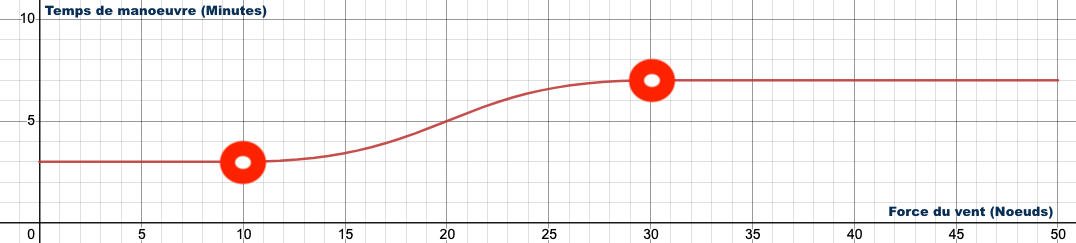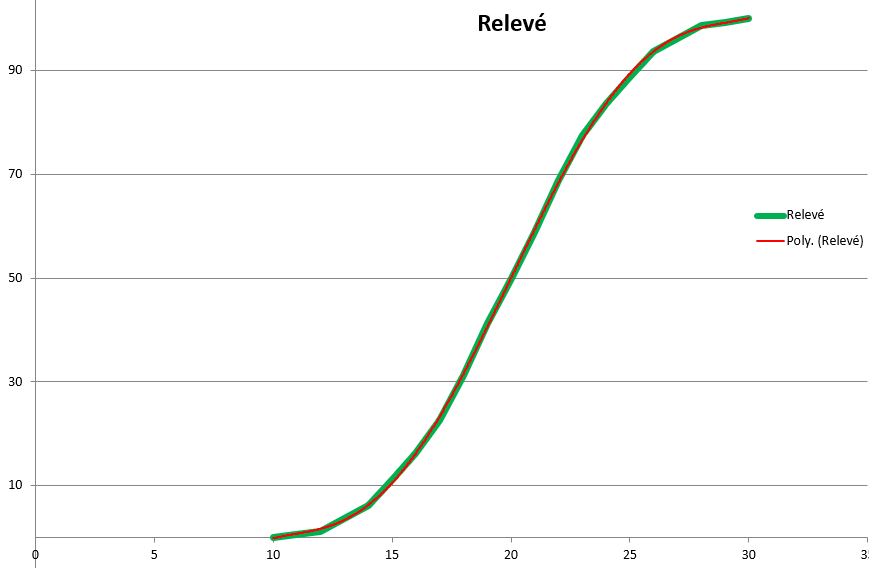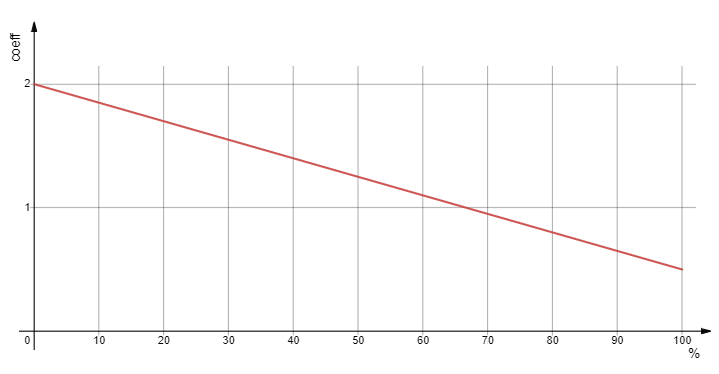### Penalty times computation

As in reality, the maneuvers and sail changes times are variable in Virtual Regatta, depending on the force of the wind and the boat used.

On this page , we find the different maneuvering times for each boat as well as the following curve :On global way, the maneuver time $T_m$ is equal to :

• the minimum maneuvering time $T_ {min}$ if the wind speed (TWS) is less than 10 knots,
• the maximum maneuvering time $T_ {max}$ if the wind speed (TWS) is greater than 30 knots (until exception),
• the result of the following calculation : $$T_m(tws) ={{{ {{(T_{max} - T_{min}) \times f(tws)}\over 100} + T_{min}}\times C_{stamina}}\times C_{boat}}$$ With :
• $T_m(tws)$ : Maneuver time
• $T_{min}$ : Minimum maneuvering time
• $T_{max}$ : Maximum maneuvering time
• $f(tws)$ : Normalized contribution from 0 to 100% of the reference curve
• $C_{stamina}$ : Stamina multiplier from 0,5 to 2
• $C_{boat}$ : Boat multiplier from 1 to 2

Using the curve provided by VR, we created a reference curve..

To do so, the curve provided was printed in large format on graph paper..

Then the curve values were recorded for each intersection with the mark to obtain the following table :Finally, the equation of the raised curve was approximated using a spreadsheet..

In green, the raised curve.

In red, the curve resulting from the equation.

$$f(tws) ={8,21565 \times 10^-15 \times tws^6 + 0,00025679 \times tws^5 - 0,025678992 \times tws^4 \\+ 0,956690978 \times tws^3 - 16,3150717 \times tws^2 + 130,3637174 \times tws - 397,862533 }$$

As on a real boat, the state of form of the skipper affects the time required to carry out a maneuver.

It is to take this aspect into account that Virtual Regatta has introduced the energy of the skipper (or stamina) as a parameter.

On this page, you can find the various information relating to this functionality, in particular concerning the impact of the state of form on the maneuvering time represented by this curve:We can therefore calculate the multiplier coefficient of the stamina according to the state of form of the skipper $Energie_{skipper}$ thanks to the following equation :

$$C_{stamina} ={ Energie_{skipper} \times -0,015 + 2 }$$

As on a real boat, the type of boat affects the time required to perform a maneuver.

To date, Virtual Regatta has not officially communicated the coefficient for each boat.

Nevertheless, thanks to the effort of the community, we know that there are 4 different coefficients :

$\times 1$ , $\times 1,2$ , $\times 1,5$ , $\times 2$

After testing, the table below seems to achieve consensus but cannot be guaranteed.

BoatCoefficient
Figaro 31
Class 401
Imoca1,2
Mini 6.501
Ultim (solo)1,5
Ultim (crew)1,5
Ocean 501
Caravelle2
Super Maxi 1001,5
Offshore Racer1

Thanks to these equations as well as to the reference values ​​of the various maneuvers contained in the polars of the game, ITYC.fr is able to calculate for each 1 / 10th of a knot of wind, the value of the penalty that will be applied for all types of boats.